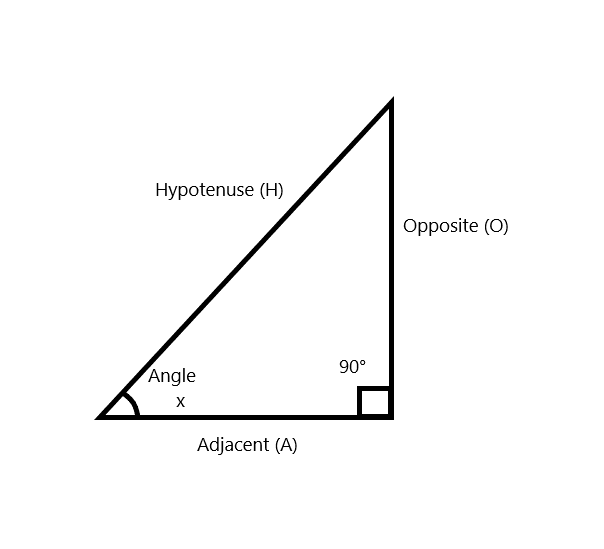# Precalculus: trigonometry questions and answersRecent questions in Trigonometry
Trigonometry
ANSWERED### Please, help to find a model for simple harmonic motion satisfying the specified conditions. Displacement (t = 0) 0, Amplitude 3 meters, Period 6 seconds.

Trigonometry
ANSWERED###Find the Opposite Side (O) of the triangle if the Adjacent Side (A) is 18 and the Hypotenuse (H) is 47.

Trigonometry
ANSWERED### Evaluate the logarithmic expression without using a calculator. $$\displaystyle{\log{{100}}},{000}$$.

Trigonometry
ANSWERED### Find the values of the trigonometric functions of θ from the information given. $$\displaystyle{\cot{ Trigonometry ANSWEREDasked 2021-09-11 ### \(\displaystyle{\sin{{x}}}+{\sin{{3}}}{x}={0}$$

Trigonometry
ANSWERED### A Major League baseball diamond has four bases forming a square whose sides measure 90 feet each. The pitcher’s mound is 60.5 feet from home plate on a line joining home plate and second base. Find the distance from the pitcher’s mound to first base. Round to the nearest tenth of a foot.

Trigonometry
ANSWERED### Find the area of the shaded region. $$\displaystyle{r}^{{2}}={\sin{{\left({2}\theta\right)}}}$$

Trigonometry
ANSWERED### Please, rewrite the expression as an algebraic expression in x. $$\sin(\tan^−1x)$$

Trigonometry
ANSWERED### Prove that: $$\displaystyle{\left({1}+{\frac{{{1}}}{{{{\tan}^{{2}}{A}}}}}\right)}{\left({1}+{\frac{{{1}}}{{{{\cot}^{{2}}{A}}}}}\right)}={\frac{{{1}}}{{{{\sin}^{{2}}{A}}-{{\sin}^{{4}}{A}}}}}$$

Trigonometry
ANSWERED### Use the Half-angle Formulas to find the exat value of each expression. $$\displaystyle{{\tan{{22.5}}}^{\circ}}$$

Trigonometry
ANSWERED### Find $$\displaystyle\frac{1}{{ \sec{\theta}- \tan{\theta}}}+\frac{1}{{ \sec{\theta}+ \tan{\theta}}}$$

Trigonometry
ANSWERED### Two direction angles of a vector are given. Find the third direction angle, given ths either obtuse $$\displaystyle\alpha={60}^{\circ},\beta={50}^{\circ},\gamma\ \text{ is obtuse}$$

Trigonometry
ANSWERED### Two direction angles of a vector are given. Find the third direction angle, given that is either acute. $$\displaystyle\alpha={\frac{{\pi}}{{{3}}}},\gamma={\frac{{{2}\pi}}{{{3}}}},\beta\ \text{ is acute}$$

Trigonometry
ANSWERED### Solve the equation on the indicated interval $$\displaystyle{\tan{\theta}}-{\sec{\theta}}={1}\quad{\left[{0}^{\circ},{360}^{\circ}\right]}$$

Trigonometry
ANSWERED### Let P(x, y) be the terminal point on the unit circle determined by t. Then $$\sin t = , \cos t = ,\ \text{and}\ \tan t =$$.

Trigonometry
ANSWERED### Prove the equation: $$\displaystyle{\frac{{{\tan{{x}}}}}{{{\tan{{x}}}+{\cot{{x}}}}}}={{\sin}^{{2}}{x}}$$

Trigonometry
ANSWERED### If $$\displaystyle{\tan{{x}}}={\frac{{{b}}}{{{a}}}}$$, then find the value of $$\displaystyle\sqrt{{{\frac{{{a}+{b}}}{{{a}-{b}}}}}}+\sqrt{{{\frac{{{a}-{b}}}{{{a}+{b}}}}}}$$

Trigonometry
ANSWERED### Prove that $$\displaystyle{2}{\arcsin{{\left({x}\right)}}}={\arccos{{\left({1}-{2}{x}^{{2}}\right)}}}$$ for $$\displaystyle{x}\in{\left({0},{1}\right)}$$

Trigonometry
ANSWERED### Verify identify $$\displaystyle{\csc{{\left({x}\right)}}}{\sin{{\left({2}{x}\right)}}}{{\sec}^{{2}}{\left({x}\right)}}={\frac{{{2}}}{{{\cos{{\left({x}\right)}}}}}}$$

Trigonometry
ANSWERED